# 06-1350/Class Notes for Tuesday October 3

(diff) ← Older revision | Latest revision (diff) | Newer revision → (diff)
Jump to: navigation, search

### Attempted solution of the exercise

I'm talking about the one stating that

Z{Ribbon knots} = {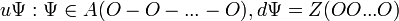$u\Psi: \Psi \in A(O-O-...-O), d\Psi = Z(O O...O)$}

my dumbbel didn't come out perfectly, and there might be further ugliness due to my lack of latex ability- I apologize

The inclusion of the left in the right is obvious. We're trying to do the reverse one.

Assume that we have a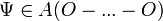$\Psi \in A(O-...-O)$ such that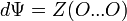$d\Psi=Z(O...O)$.

What we need to show is that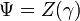$\Psi=Z(\gamma)$ for some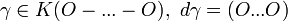$\gamma \in K(O-...-O),~ d\gamma=(O...O)$.

To do this, we first prove that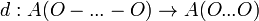$d:A(O-...-O) \to A(O...O)$ is (almost) injective.

Let's just do this for a simple (two circles) dumbbell, it will generalize to longer dumbbells easily.

Injectivity means that for some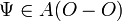$\Psi \in A(O-O)$, if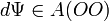$d\Psi \in A(O O)$ is zero on all invariants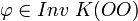$\varphi \in Inv ~ K(O O)$, then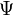$\Psi$ has to be zero on all invariants in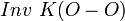$Inv ~ K(O-O)$.

We use that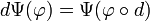$d \Psi ( \varphi)=\Psi ( \varphi \circ d)$

The key here is that the edge connecting the two circles carries no topological information, it's contractible. Therefore, an invariant of the knotted dumbbell cannot depend on how the edge wiggles- we can contract the edge to a point and get a vedge of two knotted circles, and there's only one way to vedge circles together. Therefore, all invariants of the knottings of the dumbbell are of the form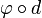$\varphi \circ d$, where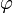$\varphi$ is an invariant of the knottings of two circles. And hence we're done.

Of course there's a lie here: we are talking about framed graphs and so the edge does carry some information: which side it's attached to on each circle, and whether it's twisted or not. So there are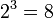$2^3=8$ preimages of each value of d. (Still a lie: If the circles are linked, I think it's countably many, given by the sides and how many times the edge is twisted, but we only care about the unlinked case, and there two twists equal nothing.)

Now, still thinking about a simple dumbbell, we can show that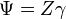$\Psi=Z\gamma$ for some knotted dumbbell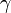$\gamma$.

We have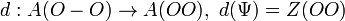$d:A(O-O) \to A(O O), ~ d(\Psi)=Z(O O)$. We know that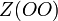$Z(O O)$ has 8 preimages, and all of those are Z-images of the dumbbells we get from the 8 different ways of attaching d (think connected sum). Therefore, one of those has to be the$\gamma$ we are searching for.

Clearly, this works just as well for the dumbbell with k circles, with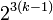$2^{3(k-1)}$ in place of 8.

And obviously, for the$\gamma$ we've found,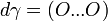$d\gamma=(O...O)$.

And we are done.

Yesterday I posted something stupid about this same problem, then realized and took it off, so I'm not sure anymore that this really works... any comments welcome!